This visualization cheat sheet is a great resource to explore data visualizations with Python, Pandas and Matplotlib. The Python ecosystem provides many packages for producing high-quality plots, graphs and visualizations.

In this guide, we will discuss the basics and a few popular visualization choices. The article starts with the basic steps for creating visualization. Next these steps are covered in detail. The end of this article has useful resources for visualizations - free books, guides, galleries.

There are summary images showing multiple visualizations at once. The goal of this guide is to help you building and customizing data visualizations.

Let's dive into visualization cheat sheet. Below you can find most popular plots from Seaborn: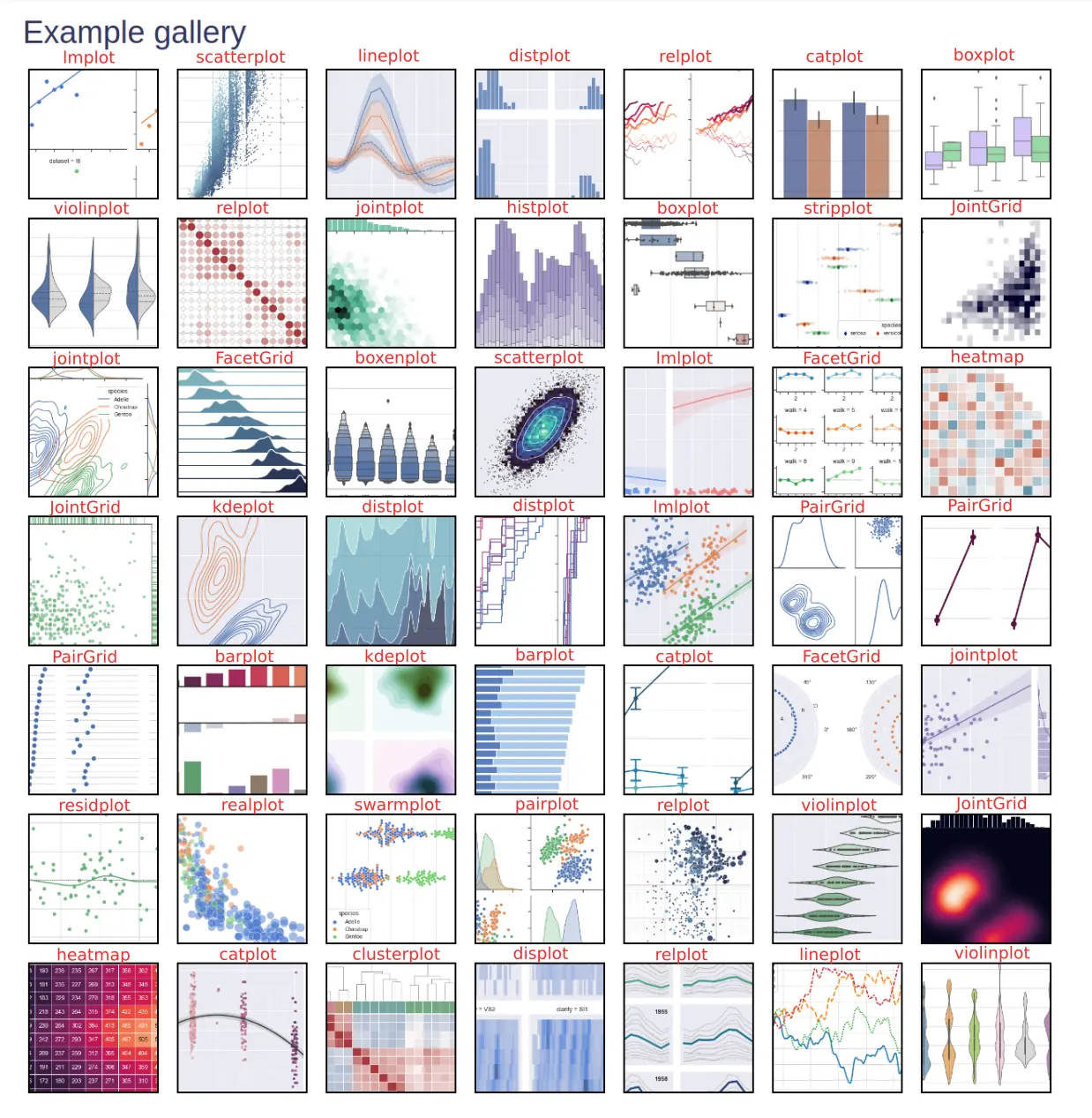## How to create good visualization

Python offers a ton of options and ways to visualize and summarize data which makes Python a natural choice for Data science.

Every great story starts with an idea. The same is with the visualization - we need idea and steps to follow to create great visualization.

1. Idea
2. Collect and select data
3. Data cleaning
4. Prepare data
1. dimensions
2. X and Y axis data
3. plot type - boxplot, line chart
5. Select tool
6. Select style and color palette
7. Customize the plot
1. title
2. labels
3. data format
4. size

## Data Setup

In this post we will use two DataFrames:

• DataFrame with random numbers
• Seaborn dataset

Creating DataFrame with 1000 numbers using normal distribution:

``````import pandas as pd
import numpy as np

ts = pd.Series(np.random.randn(1000), index=pd.date_range("1/1/2000", periods=1000))
df = pd.DataFrame(np.random.randn(1000, 4), index=ts.index, columns=list("ABCD"))
``````

result:

A B C D
2000-01-01 -0.004858 0.618783 -0.960541 -0.118617
2000-01-02 -0.476119 0.972206 0.457535 -0.099867
2000-01-03 -0.043310 0.218806 -0.751540 -0.501480
2000-01-04 -1.913368 0.143043 1.140921 -0.569990
2000-01-05 1.076793 0.809909 1.009482 0.716194

Seaborn DataFrame

``````import seaborn as sns

``````

data looks like is:

year month passengers
0 1949 Jan 112
1 1949 Feb 118
2 1949 Mar 132
3 1949 Apr 129
4 1949 May 121

## Pandas visualization cheat sheet

Pandas can visualize DataFrame by using the method `plot()`. It has a backend specified by the option `plotting.backend` - by default - `matplotlib`.

Documentation for this method is available on this link: DataFrame.plot.

### Setup, import, save

We need several imports to plot data with Python, Pandas and Matplotlib.

``````import pandas as pd
import matplotlib.pyplot as plt
``````

Save and show figure:

``````plt.savefig('plot.png')
plt.savefig('plot.png',  transparent=True) #transparent

plt.show()
``````

### Figure

To create new figure in Matplotlib with a given size:

• set size in inches
• figaspect will determine the width and height for a figure that would fit array preserving aspect ratio
``````fig = plt.figure()

fig = plt.figure(figsize=(10,5)) # size in inches

fig = plt.figure(figsize=plt.figaspect(3.0))

w, h = figaspect(2.)
fig = Figure(figsize=(w,h))
``````

### Axes

``````fig.add_axes()
fig.delaxes(ax)
``````

### Subplot

Working with Subplots in Matplotlib

``````add_subplot(nrows, ncols, index, **kwargs)

fig, axes = plt.subplots(nrows=2,ncols=2)
fig, axes = plt.subplots(nrows=4)
``````

### Matplotlib Markers

``````ax.scatter(x,y,marker= ".")
ax.plot(x,y,marker= "o")
``````

Available markers in Matplotlib:

• `"."` - point
• `"o"` - circle
• `"v"` - triangle down
• `"s"` - square
• `"D"` - diamond
• `"*"` - star marker
To find more markers we can visit: matplotlib.markers API

### Linestyles in Matplotlib

To find different line styles we can visit: set_linestyle:

• `'-'` - solid line
• `'--'` - dashed line
• `'-.'` - dash-dotted line
• `':'` - dotted line
``````x = df['A']
y = df['B']

plt.plot(x,y,linewidth=5.0)
plt.plot(x,y,linestyle= 'solid' , color='y')
plt.plot(y,x,ls= '--')
plt.plot(y,x,'--' ,x**2,y**3,'-.' )
``````

Plot different line styles with Matplotlib:

• color
• style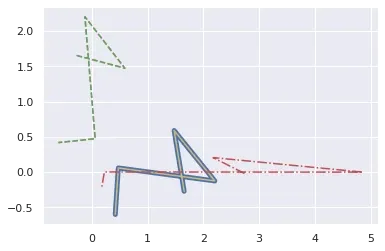``````from math import *
import numpy as np

x = np.arange(0,1.0,0.01)
y1 = np.sin(2*pi*x)
y2 = np.sin(4*pi*x)
lines = plt.plot(x, y1, x, y2)

plt.setp(lines, linewidth=2)
``````

Plot different color lines with `plt.setp`: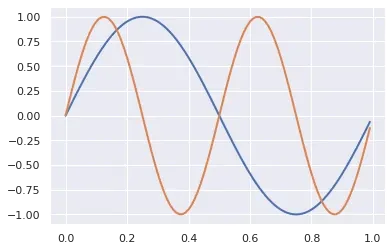``````import matplotlib.pyplot as plt
import numpy as np
import pandas as pd

cycler = plt.cycler(linestyle=['-', ':', '--', '-.'],
color=['r', 'b', 'y', 'g'])
fig, ax = plt.subplots()
ax.set_prop_cycle(cycler)
df.plot(ax=ax)
plt.show()
``````

multiple line styles and colors: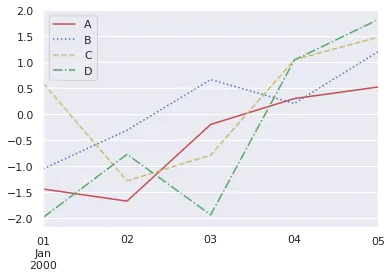### Pandas plot Series

To plot Pandas Series we can call method `plot()` directly on the Series:

``````import pandas as pd
s = pd.Series([5, 7, 2, 4, 1])
ax = s.plot(kind='bar', figsize=(10,5))
``````

The result is bar plot from the Series: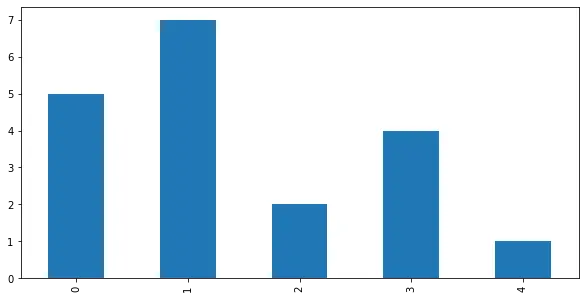### Pandas plot DataFrame

We can Plot DataFrame in Pandas by calling the method `plot()`.

``````ax = df.plot()
``````

By default all numeric columns will be used for the visualization: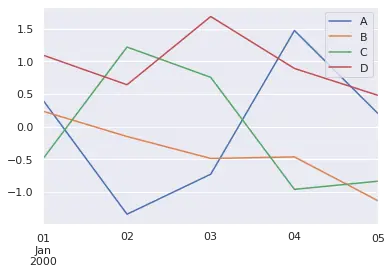Prior plotting DataFrame we can:

• select only the columns that will be plot
• set index or select X and Y data
• format and clean data

To plot DataFrame as bar plot, using the year and month as X axis with custom figure size we can do:

``````df.set_index(['year', 'month']).plot(kind='bar', figsize=(30,10))
``````

This will plot number of passengers as Y axis: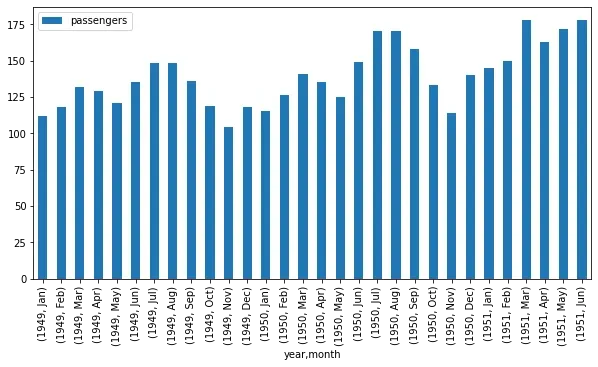### Title, Labels, Legend

``````ax.set_xlabel('Year')
ax.set_ylabel('Passenger')
ax.set_title('Passengers per year')
ax.legend(labels, loc='best')

ax.set(title= 'Title', ylabel= 'Y label',  xlabel= 'X axis')
``````

### Ticks

``````import pandas as pd
s = pd.Series([5, 7, 2, 4, 1])
ax = s.plot(figsize=(10,5))

ax.yaxis.set(ticks=range(1,9,3), ticklabels=['min', 'mid', 'max'])
ax.tick_params(axis= 'y', direction= 'out', length=5)
``````

result: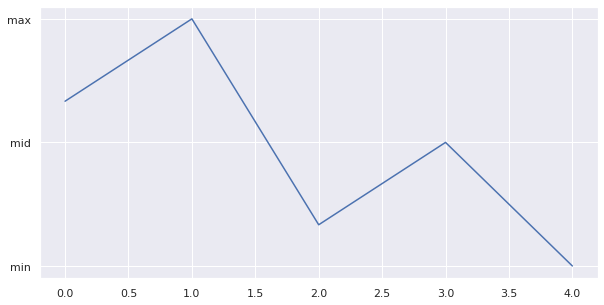### Margins, Limits

``````import pandas as pd
s = pd.Series([5, 7, 2, 4, 1])
ax = s.plot(figsize=(10,5))

ax.margins(x=0.5,y=0.5)
# ax.axis('equal') # Equal axis size
# ax.set_xlim(1,5) # set x limit
ax.set(xlim=[-1,5],ylim=[1,9]) # set x & y limits
``````

result: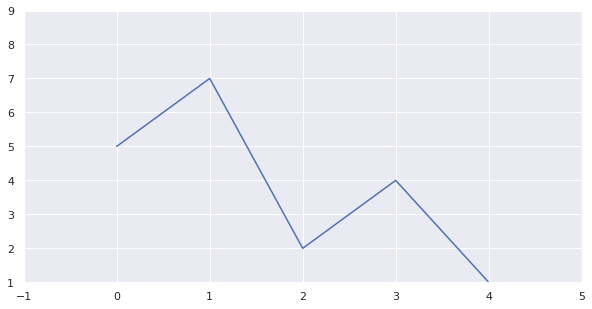### Parameters

• `x` - X axis data
• `y` - Y axis data
• `kind='bar'` - plot type
• `ax` -
• `figsize` - plot size in inches
• `subplots=True` - subplots for each column
• `sharex=False` - in case of subplots - should X axis be shared
• `layout=(3,2)` - shape of the subplots - number of rows and columns
• `title` - title of the plot
• `xticks/yticks` - values to use for the xticks/yticks
• `xlabel` / `ylabel` - name to use for the labels on x-axis/y-axis
• `fontsize=12` - font size for title
• `color='green'` - plot color
• `color = ['lightblue', 'r', 'y']`

more parameters on: plot()

Example for parameter `subplots=True`: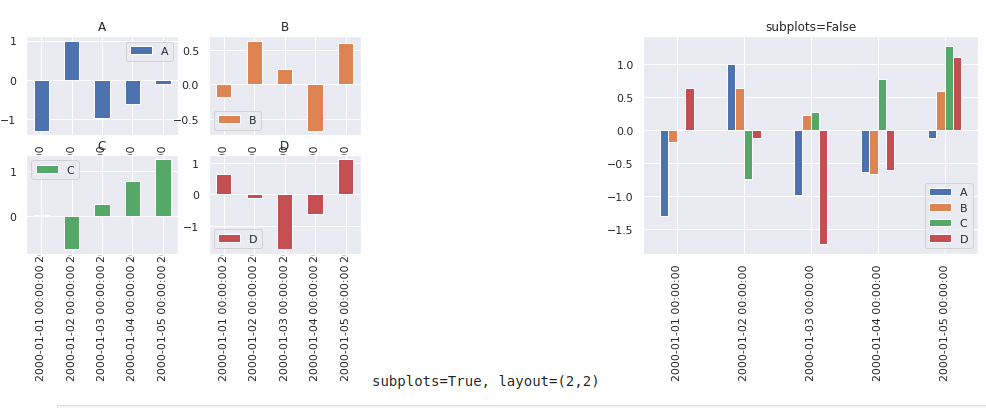### Display two plots - side by side

``````from matplotlib import pyplot as plt

# First plot
ax = plt.subplot()
plt.pie( data=df, x='A')
plt.title( 'bar' )
plt.show()

# Second plot
ax = plt.subplot()
plt.scatter( data=df, x='A', y='B' )
plt.title( 'scatter' )
plt.show()
``````

Two plots side by side - pie chart and scatter plot: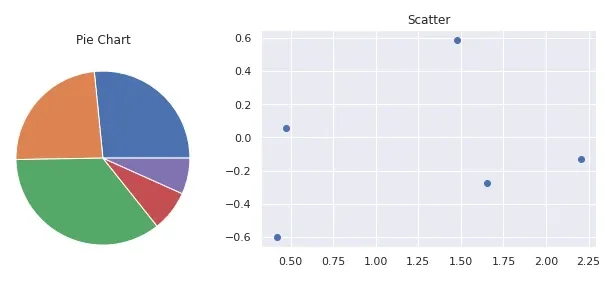### Matplotlib subplots

``````import matplotlib.pyplot as plt
import numpy as np

data = np.array([1, 4, 2, 3, 2])
plt.subplot(121)
plt.plot(data)

data = np.array([5, 7, 3, 8, 3])
plt.subplot(122)
plt.plot(data)
plt.show()
``````

result: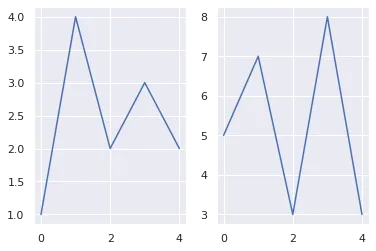### Grids of Subplots

``````for i in range(1, 5):
plt.subplot(2, 2, i)
plt.text(0.5, 0.5, str((2, 2, i)),
font size=18, ha='center')
``````

result:### Types of Pandas plots

List of the available chart types for `plot()` method. The plot type can be set as parameter - `kind`:

• `line` : line plot (default)
• `bar` : vertical bar plot
• `barh` : horizontal bar plot
• `hist` : histogram
• `box` : boxplot
• `kde` : Kernel Density Estimation plot
• `density` : same as 'kde'
• `area` : area plot
• `pie` : pie plot
• `scatter` : scatter plot (DataFrame only)
• `hexbin` : hexbin plot (DataFrame only)

Every available plot in Pandas is shown below: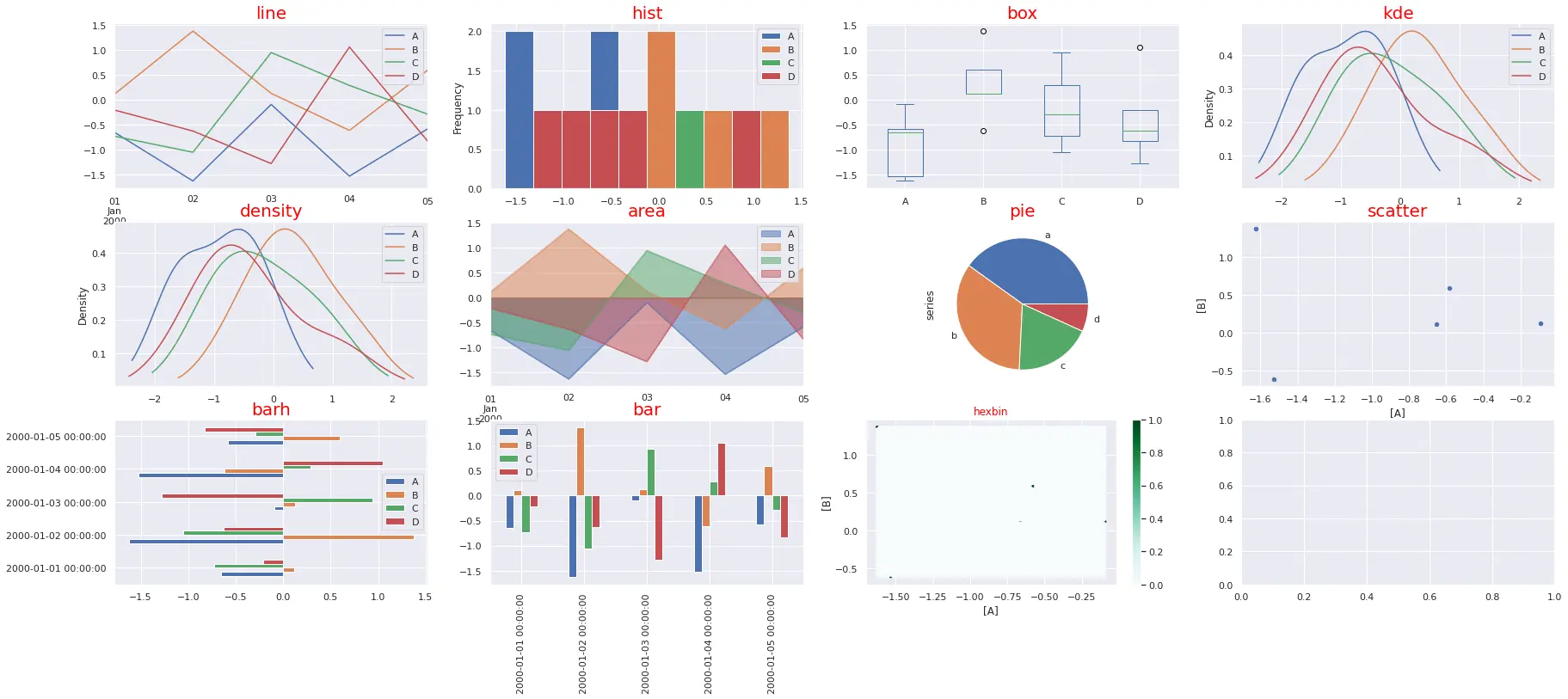The code below generates all plots:

``````import pandas as pd
import numpy as np
import matplotlib.pyplot as plt
import seaborn as sns; sns.set_theme()

ts = pd.Series(np.random.randn(1000), index=pd.date_range("1/1/2000", periods=1000))
df = pd.DataFrame(np.random.randn(1000, 4), index=ts.index, columns=list("ABCD"))

plots = [ 'line', 'hist', 'box', 'kde', 'density', 'area', 'pie', 'scatter', 'barh', 'bar', 'hexbin']

cols = df.columns
row_num = 3
col_num = 4
row_n = -1
col_n = 0

fig, axes = plt.subplots(row_num, col_num, squeeze=False, figsize=(20,14))

for ix, plot in enumerate(plots):
axes[row_n, col_n].title.set_size(20)
axes[row_n, col_n].title.set_color('red')
col_n = ix % col_num
if col_n == 0:
row_n = row_n + 1
if plot not in ['area', 'pie', 'scatter', 'hexbin']:
df.plot(kind=plot,  ax=axes[row_n, col_n], title=plot, figsize=(30,12))
elif plot == 'area':
df.plot(kind=plot,  ax=axes[row_n, col_n], title=plot, stacked=False)
elif plot == 'pie':
series = pd.Series(3 * np.random.rand(4), index=["a", "b", "c", "d"], name="series")
series.plot.pie(ax=axes[row_n, col_n], title=plot);
elif plot == 'scatter':
df.plot(kind=plot,  ax=axes[row_n, col_n], title=plot, x=['A'], y=['B'])
elif plot == 'hexbin':
df.plot(kind=plot,  ax=axes[row_n, col_n], title=plot, x=['A'], y=['B'])

plt.show()
``````

## Seaborn vs Matplotlib

Seaborn is based on Matplotlib. It enhances Matplotlib by simplifying the plot process and adding new features.

On the image below we can see all Seaborn plots like:### Seaborn setup

We can import and load datasets with seaborn by next code:

``````import seaborn as sns

``````

### Seaborn heatmap

To plot heatmap with seaborn we can do simply:

``````sns.heatmap(glue)
``````### Seaborn boxplot

Plotting boxplot in Seaborn is as easy as:

``````df_tit = sns.load_dataset("titanic")
sns.boxplot(x=df["age"])
``````

### Seaborn barplot

For barplot we need to:

• select data source
• X and Y axis - data
``````penguins = sns.load_dataset("penguins")
sns.barplot(data=penguins, x="island", y="body_mass_g")
``````

### Seaborn histogram

Seaborn histogram is called - `histplot`:

``````penguins = sns.load_dataset("penguins")
sns.histplot(data=penguins, x="flipper_length_mm")
``````

### Seaborn multiple plots

To plot multiple visualization in Seaborn side by side we can do:

``````import pandas as pd
import seaborn as sns
import matplotlib.pyplot as plt

sns.scatterplot(data=df, x='bill_length_mm', y='bill_depth_mm', hue='sex')
plt.show()

sns.scatterplot(data=df, x='flipper_length_mm', y='body_mass_g', hue='sex')
plt.show()
``````

result: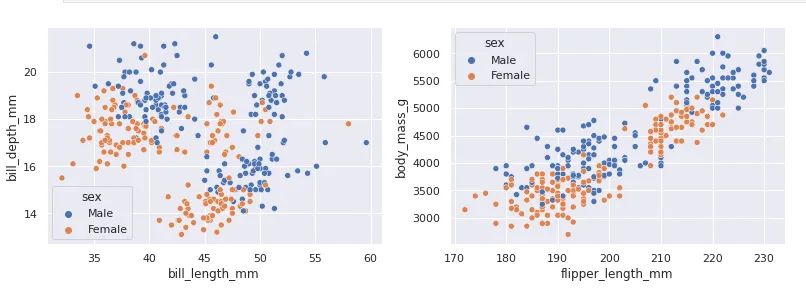## Colors

Python comes with a huge variety of named colors and palettes like the one shown below. To find the full list of colors check:

Changing colors in matplotlib:

``````color = 'red'
mpl.rcParams['text.color'] = color
mpl.rcParams['axes.labelcolor'] = color
mpl.rcParams['xtick.color'] = 'y'
mpl.rcParams['ytick.color'] = 'b'
``````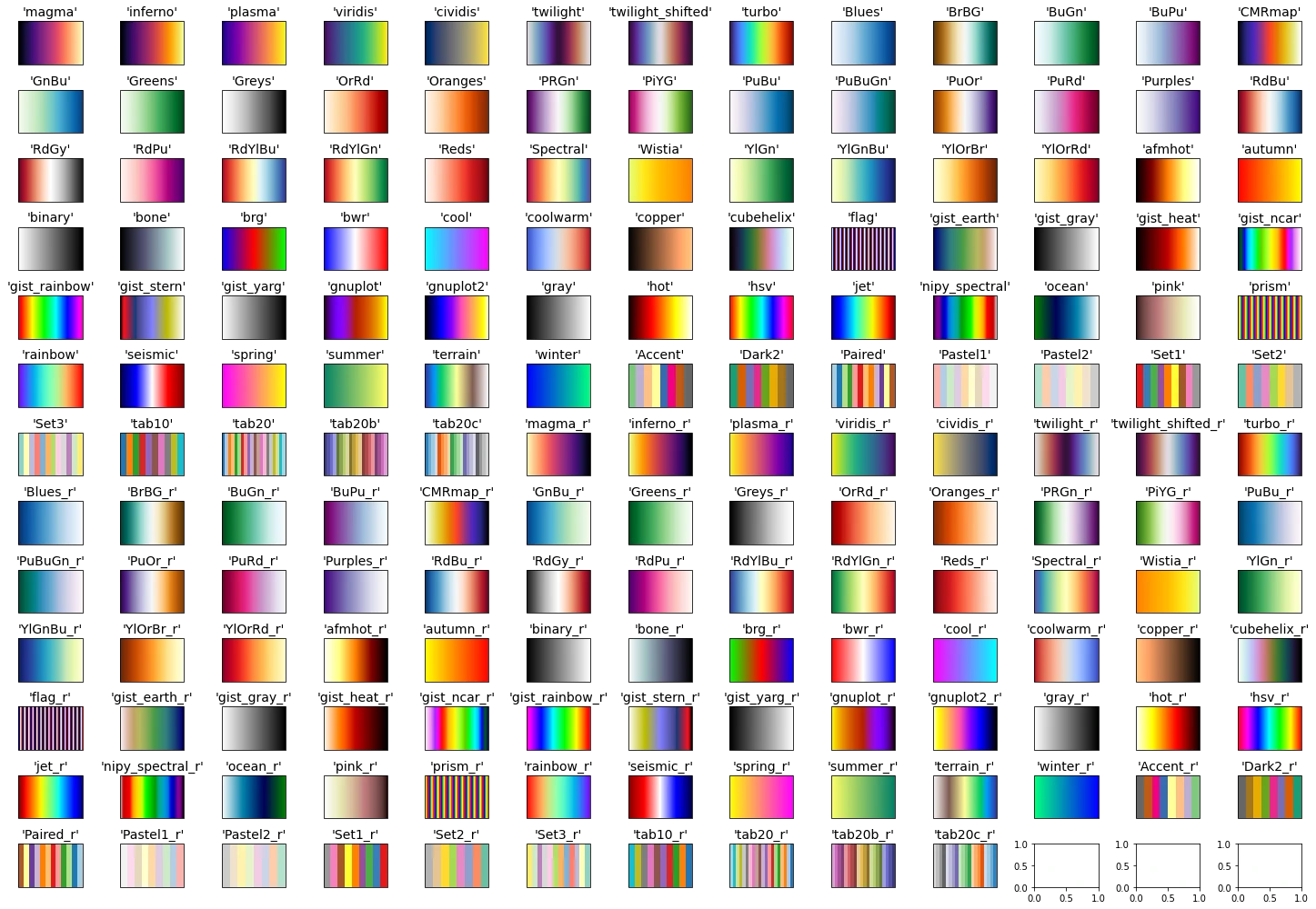## Data Visualization Libraries

• Matplotlib - most popular and widely-used plotting library
• seaborn - based on Matplotlib. High-level interface for drawing attractive and informative statistical graphics
• plotly - Plotly's Python graphing library makes interactive, publication-quality graphs.
• bokeh - interactive visualization library
• Vega-Altair - produces beautiful and effective visualizations with a minimal amount of code.
• pygal is a dynamic SVG charting library written in python
• geoplotlib - open-source Python library for visualizing geographical data.